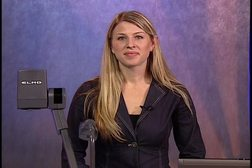PLAY PREVIEW

### Segments in this Video

#### ANOVA(07:48)

FREE PREVIEW

In this video lecture, Professor Naomi Lowe will explain analysis of variance. ANOVA uses one nominal variable with three or more levels and one continuous variable. ANOVA answers the question- are there difference between populations. ANOVA is based on estimates of population variance.

#### F-Statistic(04:14)

To calculate the F-statistics, divide the estimate between-groups population variance by the estimate within-groups population variance.

#### Hypothesis(01:26)

In ANOVA testing, the null hypothesis always predicts that the populations are equal. The alternative hypothesis predicts that the populations are not equal.

#### Logic of ANOVA(04:31)

If the null hypothesis is true it only reflects sampling error. If the alternative hypothesis is true, the variance reflects sampling error and true differences in the population. Lowe explains the logic behind F-statistic.

#### Logic of the F-Statistic(10:03)

Lowe explains the logic behind F-statistic, degrees of freedom, and how to interpret the F value, and reviews statistical notation.

ANOVA provides information on the differences between populations. Follow up tests examine which populations vary from one another and limit alpha levels.

For additional digital leasing and purchase options contact a media consultant at 800-257-5126
(press option 3) or sales@films.com.

# ANOVA: Lecture 9

Part of the Series : Statistics With Naomi Lowe
 DVD (Chaptered) Price: \$129.95 DVD + 3-Year Streaming Price: \$194.93 3-Year Streaming Price: \$129.95

Share

### Description

In this video lecture, Professor Naomi Lowe explains Analysis of Variance (ANOVA).

Length: 33 minutes

Item#: BVL150109

ISBN: 978-1-64347-561-5Closed Captioned